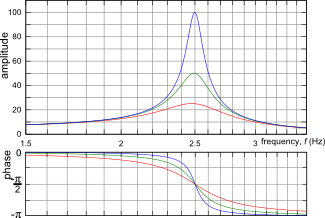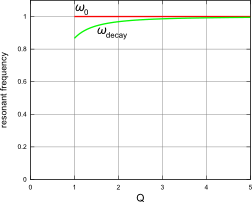There are all sorts of resonances around us, in the world, in our culture, and in our technology. A tidal resonance causes the 55 foot tides in the Bay of Fundy. Mechanical and acoustical resonances and their control are at the center of practically every musical instrument that ever existed. Even our voices and speech are based on controlling the resonances in our throat and mouth. Technology is also a heavy user of resonance. All clocks, radios, televisions, and gps navigating systems use electronic resonators at their very core. Doctors use magnetic resonance imaging or MRI to sense the resonances in atomic nuclei to map the insides of their patients. In spite of the great diversity of resonators, they all share many common properties. In this blog, we will delve into their various aspects. It is hoped that this will serve both the students and professionals who would like to understand more about resonators. I hope all will enjoy the animations.

Origins of Newton's laws of motion

History of mechanical clocks with animations
Understanding a mechanical clock with animations
includes pendulum, balance wheel, and quartz clocks

## Sunday, June 10, 2012

### Various looks of resonance curves

 All postings by author previous: 3.3 Driven Oscillations up: Contents next: 3.5 Resonance peak properties

3.4 Various looks of resonance curves↑ Fig. 10. Resonance curves for three different Q values. The resonance peaks at a phase of −π/2  the point at which the driver is the most effective at delivering power to the resonance, making the amplitude max out there. ↑ Fig. 11. A semilog plot of the same resonance. The horizontal axis is plotted on a logarithmic scale. Notice that the x-scale seems to be compressed on the right side. With semilog plots the resonance curves tend to be more symmetrical, particularly for low Q values. This is a popular method of graphing for spectrum covering several decades of frequencies, such as from 1 Hz to 1000 Hz.↑ Fig. 12. Again, the same graph plotted on log-log scales with both x and y axes having logarithmic scales. This rendition is particlarly useful if the frequency and amplitude both range over several orders of magnitude. At frequencies greater than the resonance, the amplitude approaches a straight line, falling off as amp ∝ f−2 which is a straight line when plotted on a log-log graph. The phase (not shown here) would be plotted as in Fig. 11.    This is a Bode plot for this mechanical resonance. These plots are used in electrical engineering and control theory. ↑ Fig. 13. A polar plot of the same resonance. Here the complex amplitude is plotted on the complex plane for the various values of frequency. Frequency is not shown on any axis. This is also called a parametric plot, where both the radius and angle are functions of an unshown variable, the frequency. We indicate the frequency at three places on one of the curves. The curve generally starts near the origin and positive x axis at low frequencies and progresses in a circular fashion down and finally towards the origin along the negative x axis. Most of the motion along the path occurs for frequencies near the resonant frequency.    The interesting feature is that the plots of a resonances on the complex plane makes perfect circles. The three circles are color coded the same as with the previous graphs: Q=5,10,20 for the red, green, and blue circles respectively. The magnitude of the amplitude is plotted radially. The numbers on the outer edge of the graph are the phase scale in degrees (instead of in radians as used for the phase in the previous graphs.)     This is a Nyquist plot for this mechanical resonance. These plots are used in electrical engineering and control theory.← Fig. 14. This is a logarithmic version of Figure 13, where the radial scale is logarithmic. It is useful if the magnitude of the resonance varies by several orders of magnitude. The resonances no longer make perfect circles as they do in Figure 13. The graphs on this page were made by the free professional-grade graphing software gnuplot.

Two different resonant frequencies

Above we have mentioned two different resonant frequencies, ω0 and ωdecay, given by Equation (24), which we repeat here:↑ Fig. 14A. Graph of the two resonant frequencies discussed in this posting, ω0 and ωdecay. Here we assume ω0 = 1.↑ Fig. 14B. Fractional difference (plotted in red) between the two resonant frequencies discussed in this posting, ω0 and ωdecay. The second line (green) shows the approximate error formula. It is hard to see the red line because two curves are almost identical and the green one is on top of the red one.

We use the definition of Q from (35) above: Q = τω0/2  or τ = 2Q/ω0 . We can now write ωdecay in terms of Q:

This can be used to calculate the fractional difference between the two frequencies:

which for large Q's can be approximated as:

In Fig. 14A at the right we have a graph of the two resonant frequencies ω0 and ωdecay both plotted as a function of Q.  Fig. 14B shows Δω/ω0 plotted as a function of Q. Both graphs indicate that there is less than 0.5% difference between the two frequencies for Q greater than 5. For this reason, we (and most other scientists in the field) will generally be quite lax at distinguishing between the two, unless the application at hand requires us to distinguish between them.

Yet another resonant frequency

Careful inspection of Fig. 10 above shows that the resonance curves for lower Q (e.g. Q = 5 ) do not exactly peak at 2.5Hz even though f0 = 2.5Hz for all curves. Looking at the phase graph (lower graph in Fig. 10) we see that the phase does equal  − π/2  for all three curves at f = f0 = 2.5Hz  (where f0 = ω0/2π  and f = ω/2π ). Looking at (36) of the previous posting, we see that the imaginary term in the denominator becomes zero at ω = ω0  making the complex amplitude A totally imaginary (and negative) and the phase exactly  − π/2 .

So ... at what frequency does the resonance curve peak? Using (36) we see that the dependence of magnitude of the complex amplitude on frequency (i.e. on ω) is given by:

If it were not for the leading ω in the denominator, (43) would peak at exactly ω = ω0 . To find out the maximum value with the extra ω we take the derivative of (43) and set it equal to zero. After considerable algebra we get the result that the maximum occurs at:Oddly enough, this frequency differs from ω0  by twice as much as ωdecay  does (compare with (40) above). On the other hand, neither ωmax nor ωdecay differ much from ω0 at any reasonable Q, allowing us to use ω0 in most cases for the "resonant frequency".

 All postings by author previous: 3.3 Driven Oscillations up: Contents next: 3.5 Resonance peak properties